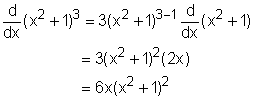Ch 2. Derivatives I Multimedia Engineering Math DerivativeFormulas TrigonometricFunctions Differentiability ChainRule ImplicitDifferentiation
 Chapter 1. Limits 2. Derivatives I 3. Derivatives II 4. Mean Value 5. Curve Sketching 6. Integrals 7. Inverse Functions 8. Integration Tech. 9. Integrate App. 10. Parametric Eqs. 11. Polar Coord. 12. Series Appendix Basic Math Units Search eBooks Dynamics Fluids Math Mechanics Statics Thermodynamics Author(s): Hengzhong Wen Chean Chin Ngo Meirong Huang Kurt Gramoll ©Kurt GramollMATHEMATICS - THEORY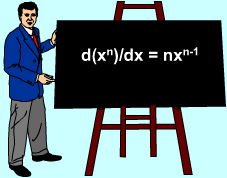Derivative Formula In previous sections, various formulas have been introduced to calculate derivatives. However, it is not possible to have a formula for every possible situation. Thus, basic formulas like d(xn)/dx = nxn-1 need to be generalized so they can be used in a variety of cases. Suppose y = (x2 + 1)3, how can dy/dx be calculated using derivative formula? Let f(u) = u3, where u = g(x) = x2 + 1. Then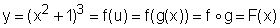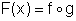The derivative of f and g can be calculated according to derivative formula. A rule is needed to calculate the derivative of F which is a composite function. This rule is named as Chain Rule. Chain Rule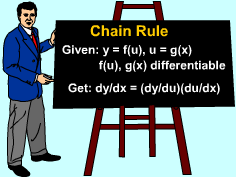Chain Rule The Chain Rule states: If the derivatives of g(x) and f(g(x)) exist, and F = f g is the composite function defined by F(x) = f(g(x)), then the derivative of F(x) exists and is given by      F'(x) = f '(g(x))g'(x) In other words, If both y = f(u) and u = g(x) are differentiable functions, then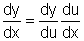The Power Rule and the Chain Rule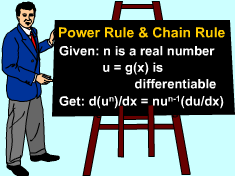The Power Rule and the Chain Rule When y = (x2 + 1)3, y relates to the power function of x2 + 1, this is a special case of chain rule. If n is any real number and u = g(x) is differentiable, then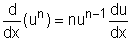Returning to the example y = (x2 + 1)3, dy/dx can be easily be determined. Let y = f(u) = u3 and u = g(x) = x2 + 1, thenso